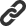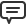# Blog

All Blog Posts  |  Next Post  |  Previous Post

#Introducing functional derivatives is easy

Bookmarks:

#### Monday, March 20, 2017

The unique feature of Analytics library is symbolic derivatives calculation. The library provides the simplest way to get a symbolic derivative expression for almost any complicated expression. There are predefined derivative for all standard functions: trigonometric, hyperbolic, logarithmic and exponential, inverse trigonometric and hyperbolic, and other.

Another fine thing is that any developer can easily define derivative for any other function. Let us consider how to define derivative for special Gamma function (https://en.wikipedia.org/wiki/Gamma_function#General). Its derivative is defined via another special function – Digamma (Polygamma function of order 0, https://en.wikipedia.org/wiki/Polygamma_function). Hereafter the Gamma function denoted as ‘G’ and the Digamma (and Polygamma) as ‘Y’. Then, the derivative formula is: d(G(x))/dx = G(x)*Y(x).

To introduce new functional derivative new class must be implemented. In our case, the class is inherited from ‘TSimpleFunctionalDerivative’ because the function has one argument only. Here is the code of the realized class:

```TGammaDerivative = class sealed(TSimpleFunctionalDerivative)
protected
function GetFunctionName(): string; override;
function BaseDerivative(argument: TBaseExpression): TBaseExpression; override;
public
class function IsRealized: boolean; override;
end;

begin
result:= 'G';
end;

begin
result:= true;
end;

var
ge: TBaseExpression;
dge: TBaseExpression;
begin
ge:= TFunctionExpression.CreateSimple('G', argument);
dge:= TFunctionExpression.CreateSimple('Y', argument);

result:= TProductExpression.MakeProduct(ge, dge);
end;
```
As can be seen from the code, there are only three methods to override. The GetFunctionName method defines that the derivative is for function with name ‘G’. The IsRealized functions returns ‘true’, that means the class is totally realized and can be used by the Analytics system. And the last method defines the derivation algorithm itself. Namely, it creates the Gamma and Digamma functions with the same argument and, according to the derivative formula above, their product.

Now we can calculate derivatives for expressions with the Gamma function. For the simplest case with input string ‘G(x)’ we get the expected output string ‘G(x)*Y(x)’. No surprise here, because we just wrote the code, which produces the output.

But the amazing thing is when we trying to calculate the derivative expression for the Gamma function with a composite argument. As example, for the input string ‘G(x^2+1)’ it produces the output string ‘(G(x^2+1)*Y(x^2+1))*(2*x)’ which is totally correct for this case. And the output is correct for all the expressions with the Gamma function. It is because the Analytics library cares about other things, like implementing the ‘chain rule’ for derivation process.

The source code of the example project is available here.

Nancy Lescouhier

Bookmarks: